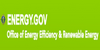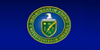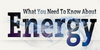### gigawatt<radiobiology> Unit of power equal to 10^9 watts, 1000 megawatts, or 1 million kilowatts. 1 gigawatt is a typical size for a nuclear fission reactor, and is expected to be the typical size of a fusion reactor. ... (09 Oct 1997) ...
Found on http://www.encyclo.co.uk/local/20973

### Gigawatt(GW) A unit of power equal to 1 billion Watts; 1 million kilowatts, or 1,000 megawatts.
Found on https://energy.gov/eere/energybasics/articles/glossary-energy-related-terms

### GigawattA unit of measure for electrical energy equal to 1 Billion watts. Gigawatts are the preferred measure of utility scale power plants. A Gigawatt is enough electricity to power 5000 homes for a year.
Found on https://www.dasolar.com/solar-energy/glossary

### GigawattOne billion watts, a watt being a unit of measure of power, or how fast energy is used. Gigawatts are typically used to describe very large quantities of power, such as the power carried by a major section of the U.S. electrical grid.
Found on http://needtoknow.nas.edu/energy/glossary/
No exact match found